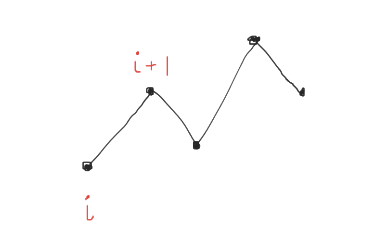ZCO-2019 UPDOWN

Hello!
PROBLEM
I am troubling understanding how the “dp” relation came.
I know there is “old editorial” for this problem but i can’t understand it.
I checked other people solutions and they are doing differently.
Can someone explain me this dp relation origin?

for(int i = n - 1; i > 0; i--){
if(arr[i] <= arr[i + 1]){ // If arr[i] shows length of largest UD starting from arr[i]
dp[i] = dp[i + 1] + 1;
}
if(arr[i] >= arr[i + 1]){ // If arr[i] shows length of largest DU starting from arr[i]
dp[i] = dp[i + 1] + 1;
}
}

Lets define

Up-Down[i] = maximum length of Up-Down Sequence starting at i

( Up-down sequence is sequence which starts with up , then down , then up … )

Down-Up[i] = maximum length of Down-Up Sequence starting at i

( Down-Up sequence is sequence which starts with Down , then Up , then Down … )

Now we want to find the up-down[i] :up-down starting at i = down-up starting at (i+1) + 1

( +1 because we can also add ith node to our sequence )

But there is one condition for the sequence starting from i to be a updown that a[i] <= a[i+1]

Hope you understood how we calculate updown[i]
Similarly we calculate downup[i]

Here is the image :Try to find out the recurrence here and also the condition

1 Like

Oh, Thanks!